## 1. 神经科学中的人工神经网络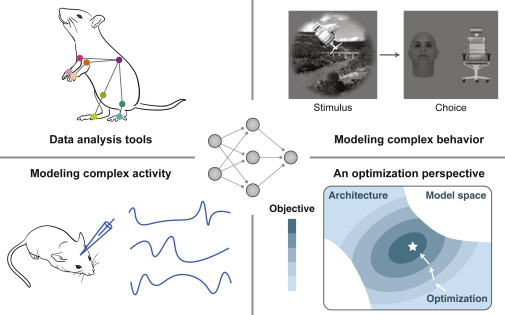（左上）神经/行为数据分析。人工神经网络可用作图像处理工具，实现高效的姿态预测。1
（右上）对复杂行为进行建模。人工神经网络可以执行涉及具有挑战性的自然视觉物体的物体辨别任务。2
（左下）说明人工神经网络可用于模拟复杂的神经活动/连接模式（蓝线）。
（右下）从优化角度理解神经回路。在此视图中，函数神经网络（星号）是目标函数在受神经网络架构（彩色空间）约束的模型的抽象空间中优化（箭头）的结果。

## 2. ANN 的基本成分和变化

### 2.1 基本成分：学习问题、体系结构和算法

#### 网络体系结构

ANN 具有难以置信的多功能性，包括广泛的架构。在所有架构中，最基本的架构是 MLP（图2A）。MLP 由多层神经元组成，其中第 l 层的神经元仅接收来自第 (l−1) 层的输入，并且仅投射到第 (l+1) 层。

$$r^{(1)}=x \tag{1}$$

$$r^{(l)}=f(W^{(l)}r^{(l-1)}+b^{(l)}),\ 1<l<N \tag{2}$$

$$y=f(W^{(N)}r^{(N-1)}+b^{(N)}) \tag{3}$$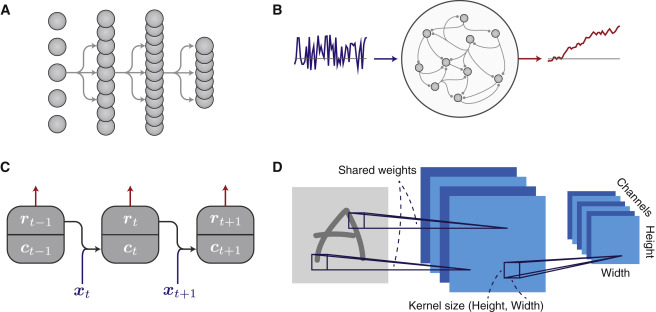（A） 多层感知器（MLP）。
（B） 递归神经网络（中间）接收输入流（左侧）。训练后，输出单元（右）应产生期望的的输出。4
（C） 递归神经网络作为前馈系统在时间上展开，每个层在一个时间步长对应于网络状态。 $c_t$ 和 $r_t$ 分别描述了时间 $t$ 的网络状态和输出活动。 $c_t$ 是 $r_{t-1}$ 和输入 $x_t$ 的函数。

（D） 用于处理图像的卷积神经网络。每层包含多个通道（第 1 中有四个，第 2 层中有六个）。通道（由正方形表示）由空间组织的神经元组成，每个神经元接收来自具有相似空间偏好的神经元的连接。这些连接的空间范围由内核大小来描述。5

#### 训练算法

$$L_{batch}=\frac{1}{M}\sum_{k∈\mathbb{B}}L(y^{(k)},y^{(k)}_{target}) \tag{4}$$

$$Δθ=−η\frac{∂L}{∂θ} \tag{方程 5}$$

$W$ 和 $b$ 等参数通常是可训练的。其他参数由建模者设置，称为超参数，例如学习率 $η$。计算梯度的一个关键要求是可微性，即模型中函数的导数定义良好。

$$y=Wx+b，或等价方程，y_i=\sum_{j}W_{ij}x_{j}+b_i \tag{6}$$

$$\frac{∂L}{∂W_{ij}}=\sum_{k}\frac{∂L}{∂y_k}\frac{∂y_k}{∂W_{ij}}=\frac{∂L}{∂y_i}x_j \tag{7}$$

$$\frac{∂L}{∂W}=\frac{∂L}{∂y}x^⊺ \tag{8}$$

$$L=\frac{1}{2}\Vert y−y_{target}\Vert^2=\frac{1}{2}\sum_j(y_j−y_{target},\ j)^{2} \tag{9}$$

$$\frac{∂L}{∂W}=(y−y_{target})x^⊺ \tag{10}$$

$$ΔW_{ij}\propto−\frac{∂L}{∂W_{ij}}=(y_{target},\ i−y_i)x_j \tag{11}$$

$$\frac{∂L}{∂r_{i}^{(l)}}=\sum_j \frac{∂L}{∂r_{j}^{(l+1)}} \frac{∂r_{j}^{(l+1)}}{∂r_{i}^{(l)}}=\sum_j \frac{∂L}{∂r_{j}^{(l+1)}}W_{ji}^{(l+1)}=\sum_j[W^{(l+1)}]_{ij}^{⊺} \frac{∂L}{∂r{j}^{(l+1)}} \tag{12}$$

$$\frac{∂L}{∂r^{(l)}}=[W^{(l+1)}]^⊺ \frac{∂L}{∂r^{(l+1)}}=[W^{(l+1)}]^⊺[W^{(l+2)}]^⊺ \frac{∂L}{∂r^{(l+2)}}=⋯ \tag{13}$$

### 2.3 网络结构的变化

#### 递归神经网络

$$c_t=W_rr_t−1+W_xx_t+b_r \tag{14}$$

$$r_t=f(c_t) \tag{15}$$

$$y_t=W_yr_t+b_y \tag{16}$$

$$r_t=f(W_rr_{t−1}+W_xx_t+b_r),\quad for\ t=1,⋯,T \tag{17}$$

$$\frac{∂L}{∂r_t}=W_{r}^{⊺}\frac{∂L}{∂r_{t+1}}=[W_{r}^{⊺}]^{2} \frac{∂L}{∂r_{t+2}}=⋯ \tag{18}$$

#### 卷积神经网络

$$r_{i_{C}i_{H}i_{W}}^{(l)}=\sum_{j_{C}j_{H}j_{W}}W_{i_{C}i_{H}i_{W},\ j_{C}j_{H}j_{W}}^{(l)}r_{j_{C}j_{H}j_{W}}^{(l−1)} \tag{19}$$

$$W_{i_{C}i_{H}i_{W},\ j_{C}j_{H}j_{W}}^{(l)}=W_{i_C,\ j_C}^{(l)}(i_H−j_H,\ i_W−j_W) \tag{20}$$

#### 激活功能

ANNs 中的大多数神经元，像它们的生物对应物一样，根据它们的输入进行非线性计算。这些神经元通常是具有单一非线性激活函数 $f(⋅)$ 的点状神经元，它将输入的总和与输出的活动联系起来。非线性对于 ANN 的功能至关重要。常用的激活函数是整流线性单元（ReLU）函数，$f(x)=max(x,\ 0)$7。ReLU 在 $x=0$ 时的导数在数学上是未定义的，但在实践中习惯性地设置为 0。ReLU 及其变体通常用于前馈网络，而双曲正切（tanh）函数通常用于递归网络 。ReLU 和类似的激活函数是不对称的，在高值时是不饱和的。尽管生物神经元在高速率下最终会饱和，但它们经常在非饱和状态下工作。因此，带有速率单元的传统神经回路模型也经常使用非饱和激活函数。

#### 归一化

$$\widehat{x_i}=γ⋅\frac{x_i−μ}{σ}+β \tag{21}$$

$$μ=\langle x_j \rangle \tag{22}$$

$$σ=\sqrt{ \langle (x_j−μ)^2 \rangle+ϵ} \tag{23}$$

### 2.4.训练算法的变体

#### 基于SGD的方法的变种

$$v^{(j)}=μv^{(j-1)}+\frac{∂L^{(j)}}{∂θ},\qquad 0<μ<1 \tag{24}$$

$$Δθ^{(j)}=−ηv^{(j)} \tag{25}$$

## 3. 构建 ANN 以解决神经科学问题的例子

### 3.1 视觉系统的卷积网络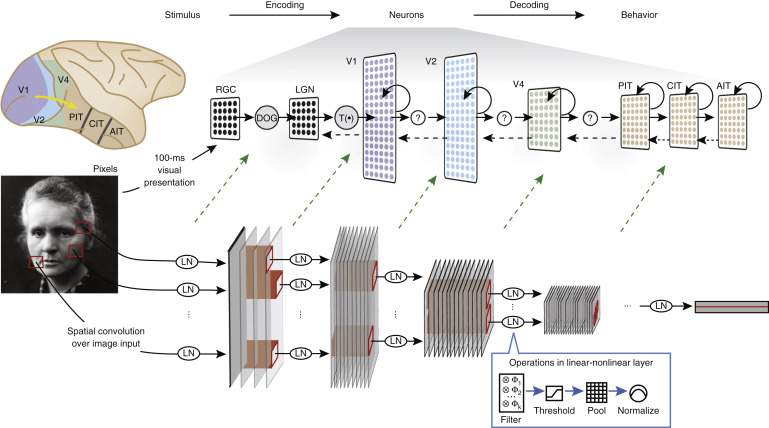### 3.2 认知和运动系统的 RNN

RNN 是处理语音和文本等序列的常用机器学习工具。在神经科学中，它们已用于建模认知、运动和导航系统的各个方面。与用于模拟视觉系统的卷积网络不同，递归网络通常针对神经科学家正在研究的特定认知或运动任务进行训练。通过比较在动物或人类执行的相同任务上训练的 RNN，可以在 RNN 和大脑之间进行并排比较。可以在多个层面进行比较，包括单神经元活性和选择性、种群解码、状态空间动力学和网络对扰动的响应。我们将在下一节详细介绍如何分析 RNN。

$$τ\frac{dr}{dt}=−r(t)+f(W_rr(t)+W_xx(t)+br) \tag{26}$$

$$r(t+Δt)≈r(t)+\frac{Δt}{τ}[−r(t)+f(W_rr(t)+W_xx(t)+br)] \tag{27}$$

$$τ\frac{dr}{dt}=−r(t)+f(W_rr(t)+W_xx(t)+w_{fb}y(t)+br) \tag{28}$$

$$y(t)=w_y^⊺r(t) \tag{29}$$

$$τ\frac{dr}{dt}=−r(t)+f([Wr+w_{fb}w_y^⊺]r(t)+W_xx(t)+br) \tag{30}$$

## 4. 分析和理解 ANNs

### 相似性比较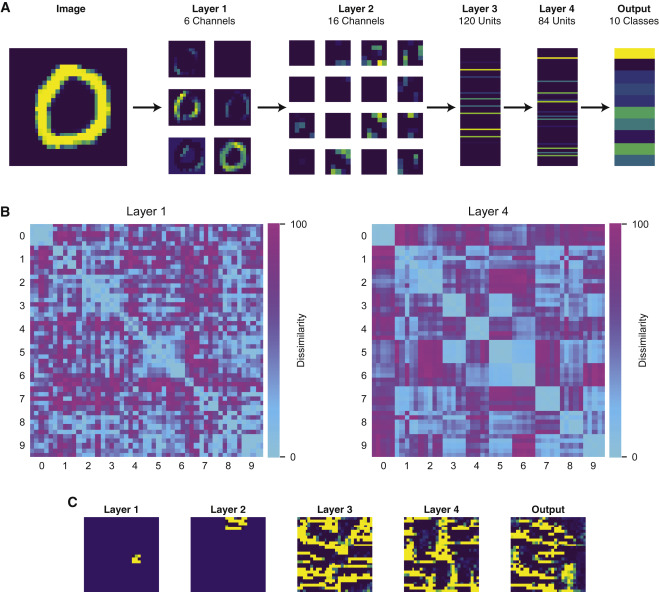(A) 在一个卷积神经网络中，对图像的神经反应被训练成手写数字的分类。该网络由两层卷积处理组成，然后是两个完全连接层。
(B) 异同矩阵（每个 D 乘 D）评估对不同输入图像的相似或不相似的神经反应。差异矩阵是针对网络的第1层和第4层的神经元计算的。 D=50。图像按类别（0、1 等）组织，每个类别有五个图像。在第4层（右）比在第1层（左）对同一类别的图像的神经反应更相似，也就是说，神经表征更基于类别。
(C) 通过基于梯度的优化，为每层的样本神经元找到首选的图像刺激。第 1 层和第 2 层是卷积的，因此它们的神经元有本地化的首选刺激。相比之下，第 3 层和第 4 层的神经元有非局部的偏好刺激。

### 复杂调谐分析

$$x→x+Δx; \quad Δx=η\frac{∂r}{∂x} \tag{31}$$

x 的空间可能维度太高（如像素空间），无法进行有效的搜索，特别是对于无梯度方法。在这种情况下，我们可以利用一个低维空间，但仍有很强的表现力。生成模型学习一个函数，将低维潜伏空间映射到高维空间，如像素空间。然后，可以在低维潜伏空间中进行搜索。

ANNs 可以用来建立复杂行为的模型，否则不容易做到，开辟了新的可能性，如研究更抽象的信息形式的编码。例如，Yang 等人，2019 年研究了任务结构的神经调谐，而不是刺激，在规则引导下的问题解决12。一个 ANN 被训练来执行动物实验中常用的许多不同的认知任务，包括感知决策、工作记忆、抑制性控制和分类。复杂的网络组织是通过训练形成的，其中递归神经元对一个子集的任务显示出选择性（图 5）。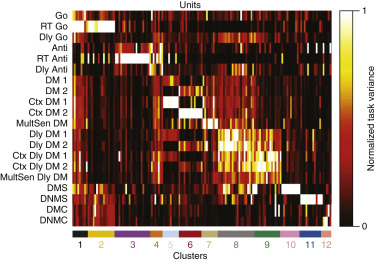### 动态系统分析

$$\frac{dr}{dt}=F(r) \tag{32}$$

$$\frac{dr}{dt}=F(r)=F(r_{ss}+Δr)≈F(r_{ss})+J(r_{ss})Δr,\quad \frac{dΔr}{dt}=J(r_{ss})Δr \tag{33}$$

$$argmin_r\Vert F(r)\Vert ^2 \tag{34}$$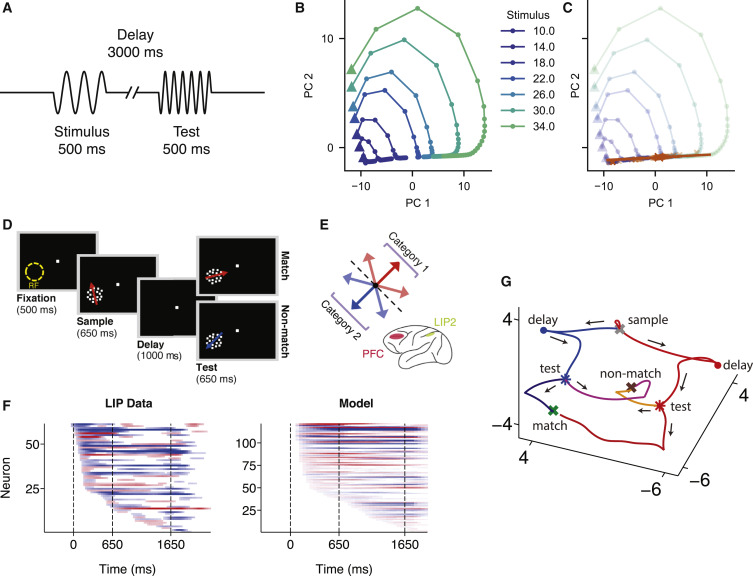（A–C）在一个简单的参数化工作记忆任务中，网络需要通过一个延迟周期（A）记忆刺激的（频率）值。网络可以通过开发线吸引子（B和C）来实现这种参数化工作记忆。
（B） PCA空间中不同刺激值的延迟期内的神经活动试验平均值。三角形表示延迟周期的开始。
（C） 通过优化找到的固定点（橙色十字）。线吸引子的方向可以通过找到对应特征值接近0的特征向量来估计。橙色线显示了围绕其中一个固定点估计的线吸引子。
（D–G）训练循环神经网络和猴子进行延迟匹配分类任务。任务是确定测试和样本刺激（视觉运动模式）是否属于同一类别（D）。这两个类别是根据刺激的运动方向定义的（红色，类别1；蓝色，类别2）（E）。在为执行该分类任务而训练的ANN中，模型的重复单元显示出类别选择性的广泛的开始时间异质性，类似于任务期间从猴后顶叶皮层（外侧顶内区域，LIP）记录的单个神经元（F）。基于 DMC 任务（G）性能的递归神经网络的神经动力学。最终决定，匹配（AA 或 BB）或不匹配（AB 或 BA）对应于位于状态空间中不同位置的不同吸引子状态。在实验数据中也发现了类似的族群活动轨迹。

## 5.生物拟真的网络架构和学习

### 5.1.结构化连接

$$\frac{dr^E}{dt}=−r^E+f_E(W_{EE}r^E−W_{EI}r^I+W_{Ex}x+b^E) \tag{35}$$

$$\frac{dr^I}{dt}=−r^I+f_I(W_{IE}r^I−W_{II}r^I+W_{Ix}x+b^I) \tag{36}$$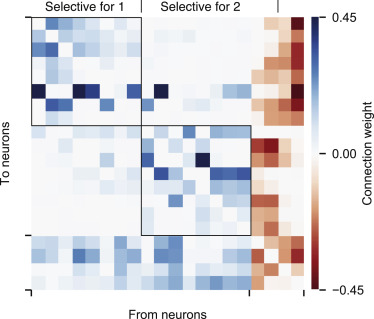### 5.2 经典计算

#### 归一化

$$r_i=f(γ \frac{I_i}{\sum_j I_j+σ}) \tag{37}$$

#### 注意

$$y=\sum_iα_iv^{(i)} \tag{39}$$

$$c_i=score \left(q,\ k^{(i)}\right) \tag{40}$$

$$α_1,⋯,α_N=normalize\ (c_1,⋯,c_N) \tag{41}$$

$$α_i=\frac{e^{c_i}}{\sum_je^{c_j}},\ 于是\ \sum_iα_i=1 \tag{42}$$

#### 门控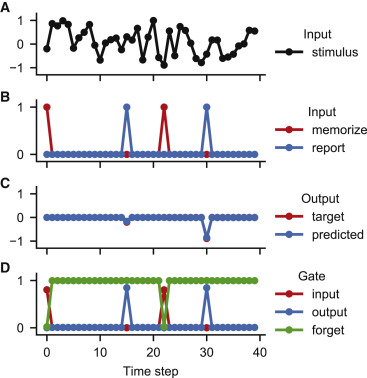（A–C）简单的记忆任务。
（A） 网络接收输入刺激流，其值在每个时间点随机且独立地采样。
（B） 当“记忆输入”（红色）激活时，网络需要记住刺激的当前值（A），并在“报告输入”（蓝色）下次激活时输出该值。
（C） 训练后，单个单元 LSTM 可以在适度的记忆时间内几乎完美地完成任务。
（D） 当记忆输入激活时，该网络打开输入门（允许输入）并关闭遗忘门（忘记以前的记忆）。当报告输入激活时，它打开输出门。

### 5.3. 学习和可塑性

#### 选择性训练和持续学习

$$L_{reg}=β\sum_{ij}M_{ij}(W_{ij}−W_{fix},\ ij)^2 \tag{44}$$

Hebbian 可塑性

$$A(t+1)=λA(t)+ηrr^⊺ \tag{45}$$

$$A_{ij}(t+1)=λA_{ij}(t)+ηr_ir_j \tag{46}$$

$$A_{ij}(t+1)=λA_{ij}(t)+f(r_i,r_j,θ) \tag{47}$$